Teachoo Questions

Class 10
Chapter 10 Class 10 - Light - Reflection and Refraction

The password to open the file is -    teachooisbest

## One student uses a lens of focal length 50 cm and another of –50 cm. What is the nature of the lens and its power used by each of them?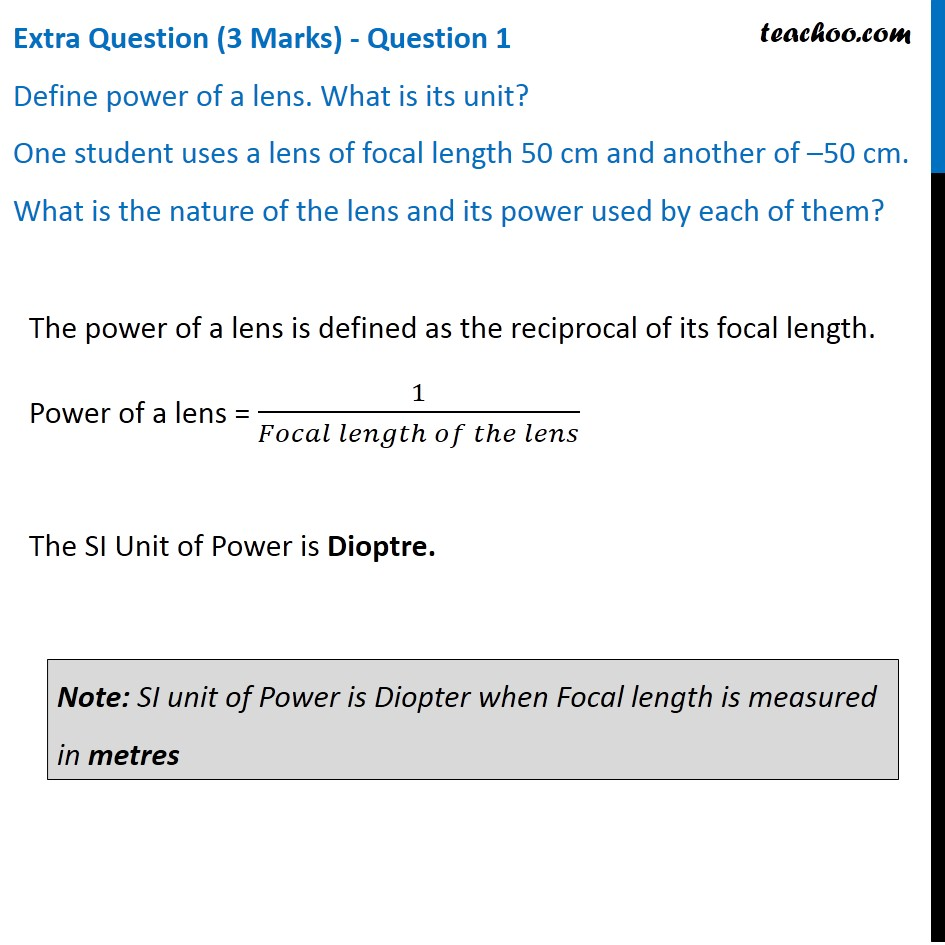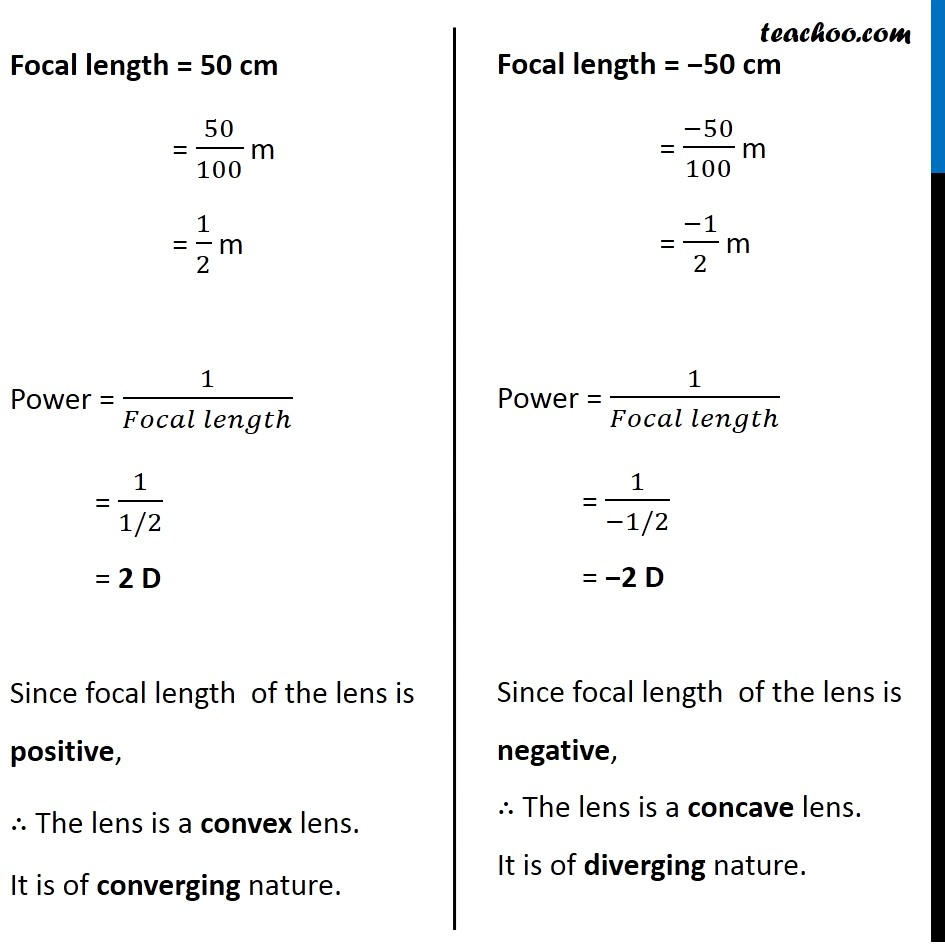## (i) Use lens formula to find the distance of the image from the lens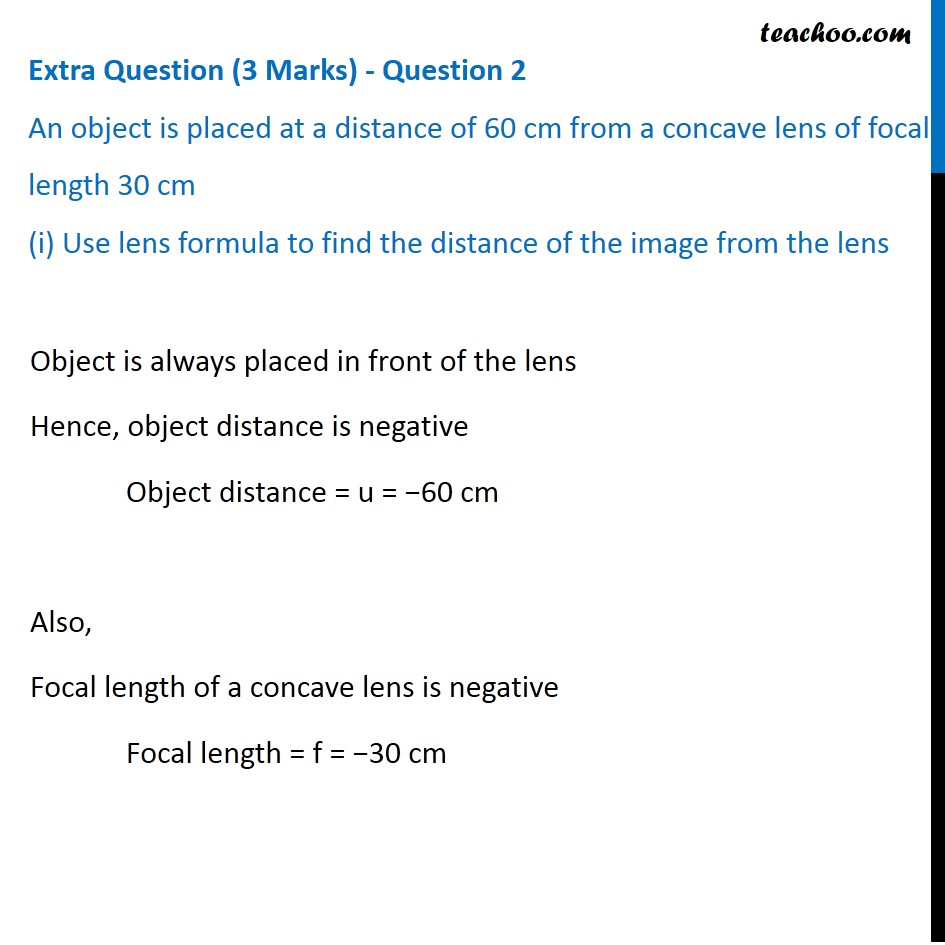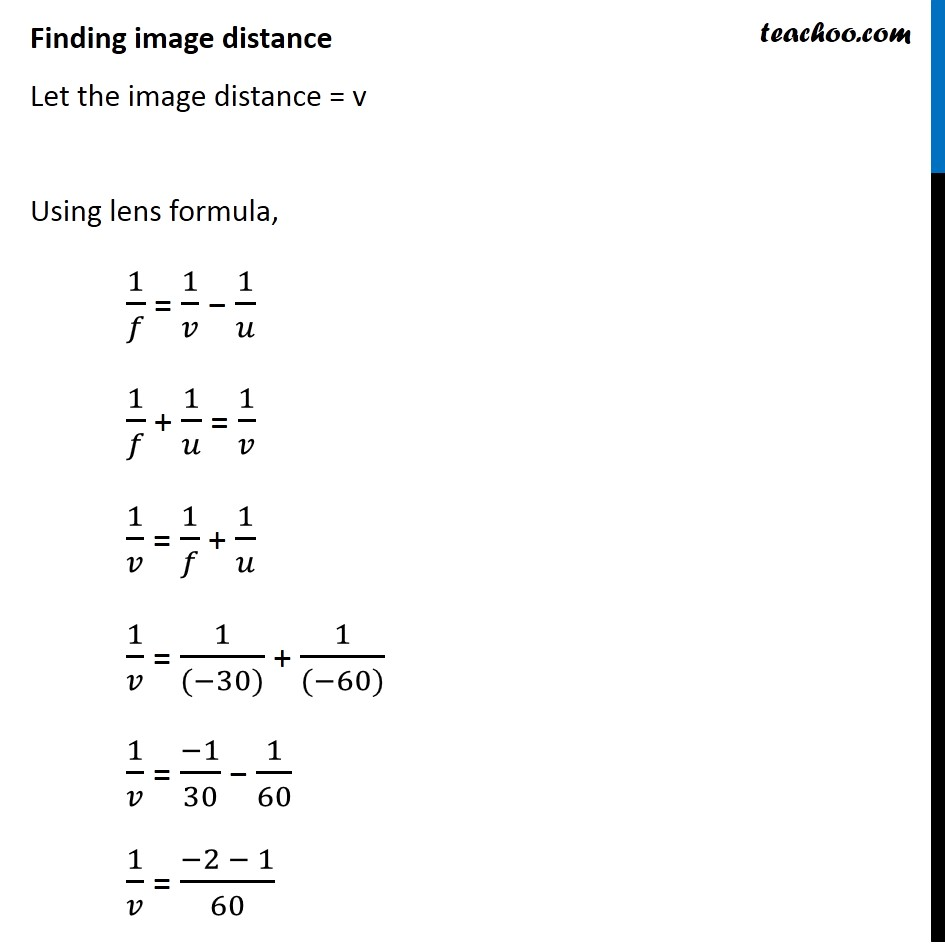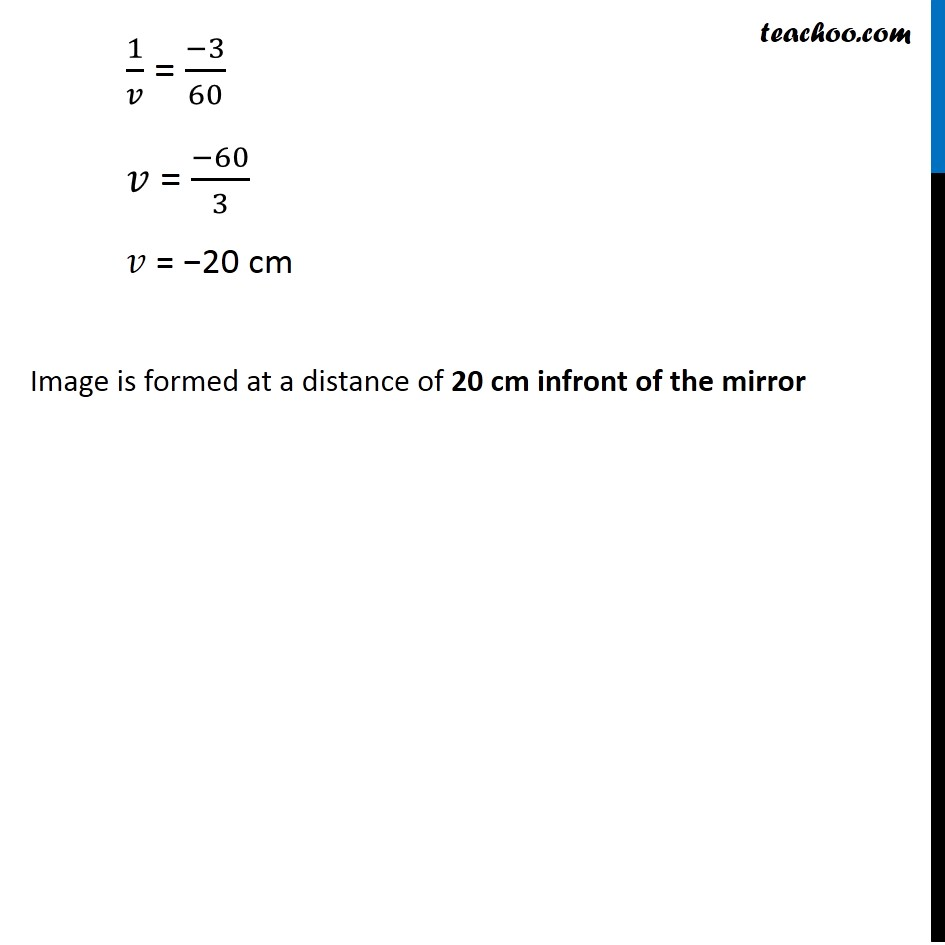## (ii) List four characteristics of the image (nature, position, size, erect/inverted) formed by the lens in this case

The image formed by the concave lens is

Virtual

Formed 20cm infront of the lens (same side as that of object)

Diminished (smaller than the size object)

Erect

## (iii) Draw ray diagram to justify your answer to part (ii)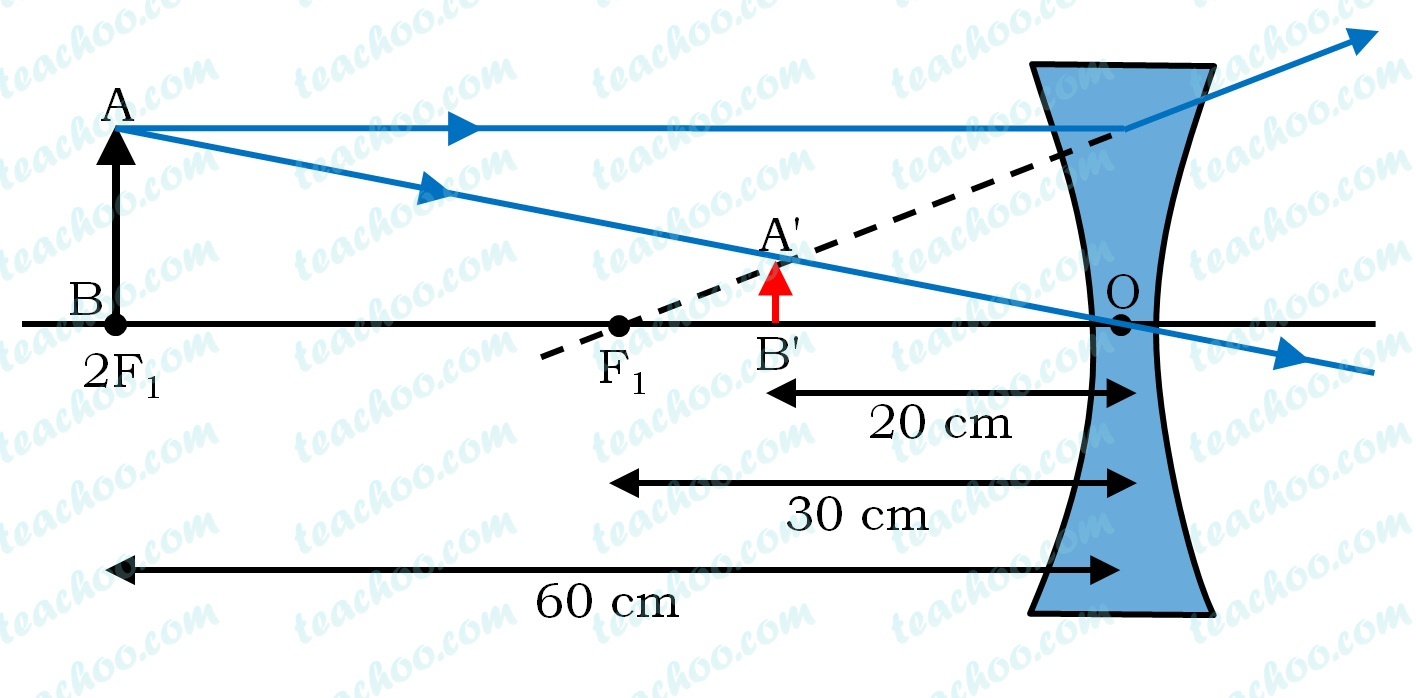## List four precautions which a student should observe while determining the focal length of a given convex lens by obtaining an image of a distant object on a screen.

The student should take the following precautions

• The lens should be held straight in a vertical position such that its faces are parallel to the screen.
• Clearest  and sharpest  image should be  obtained by adjusting  the position of lens
• At least three  observations should  be taken to get the most accurate result.
• Base  of lens,  screen and  measuring scale  should be in a straight  line.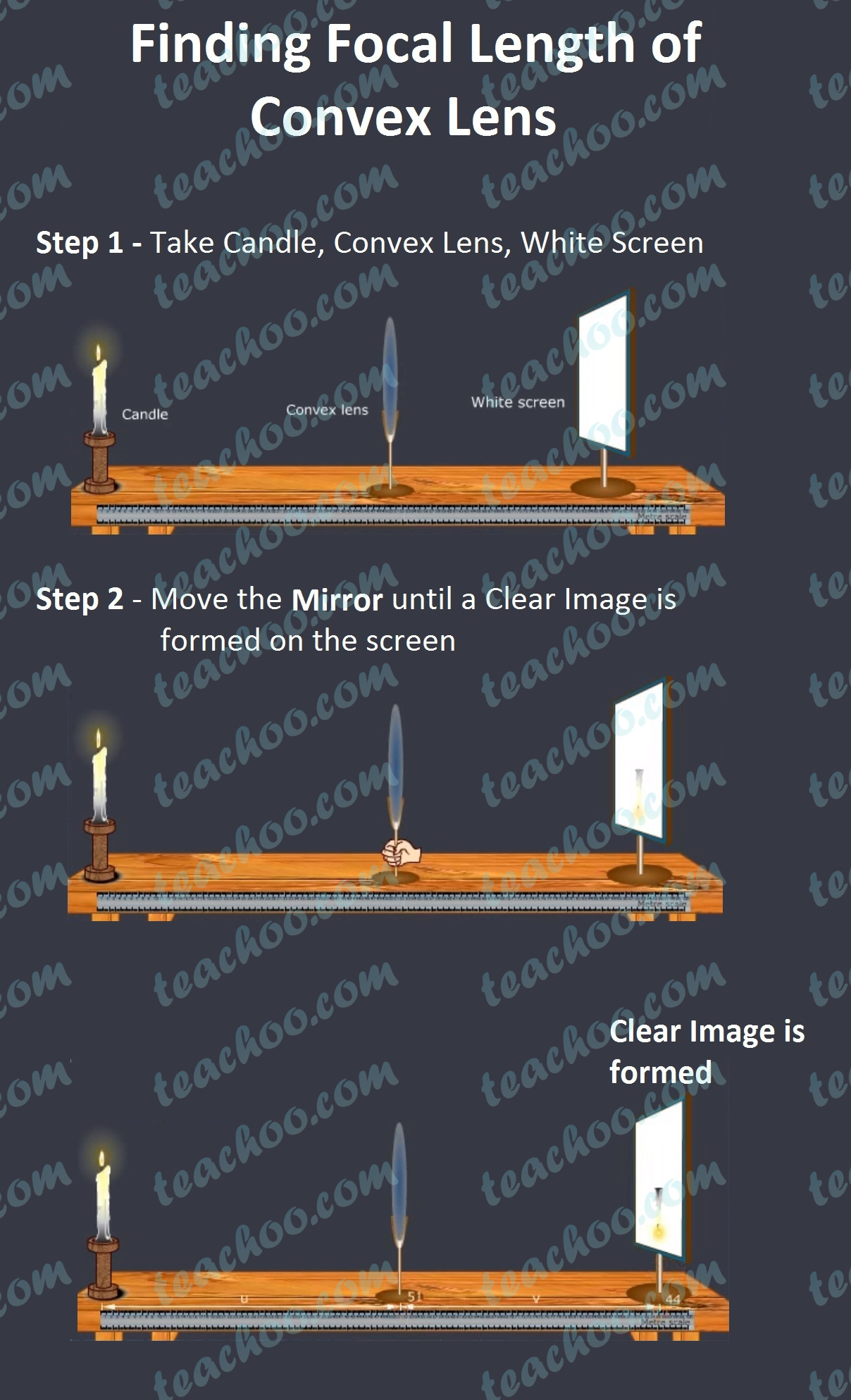## While tracing the path of a ray of light passing through a rectangular glass slab a student tabulated his observations. If in his experiment for two readings he takes two values of angle of incidence as 40 and 50, what should be the correct values of angle of emergence and angle of r in each case?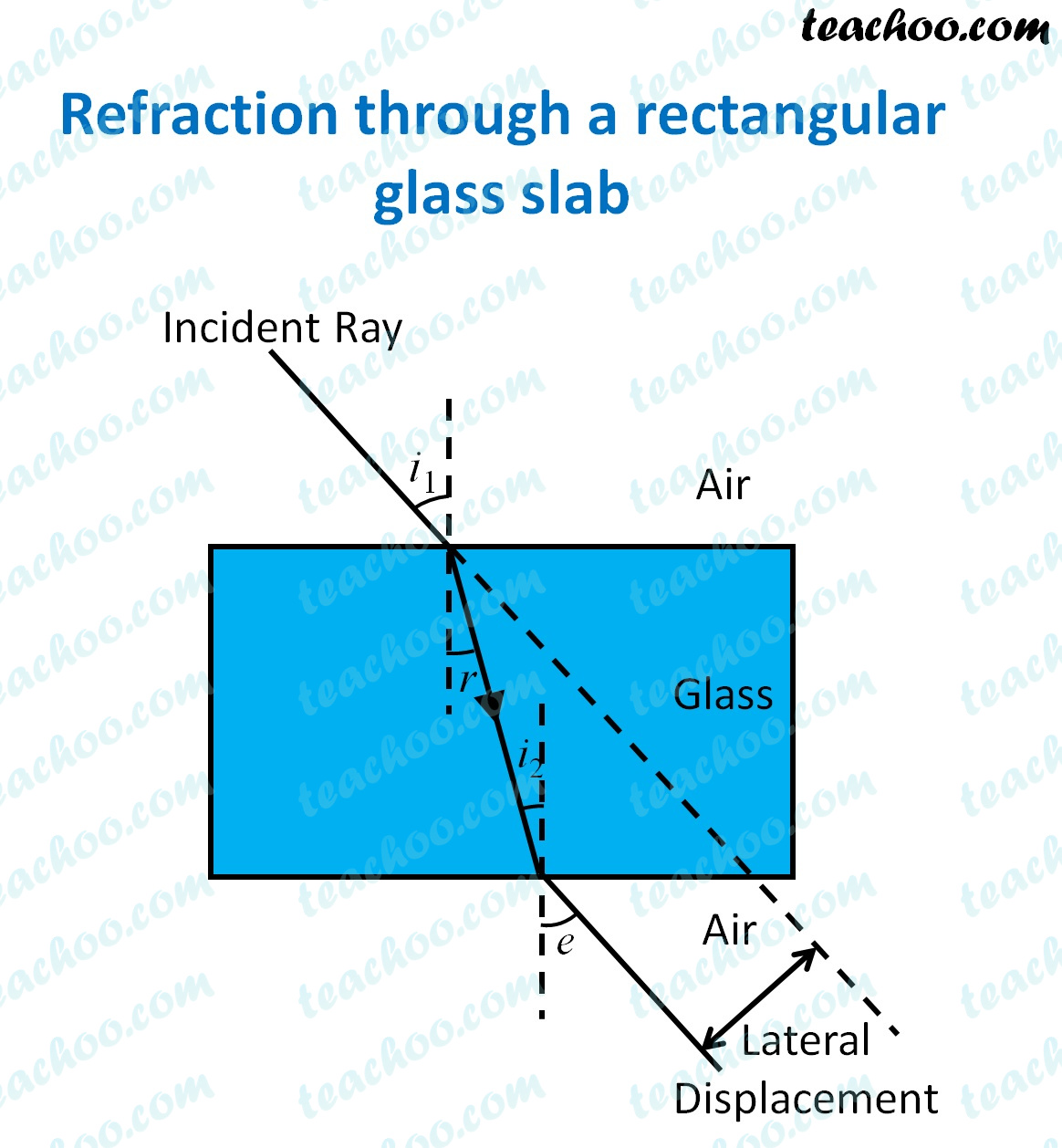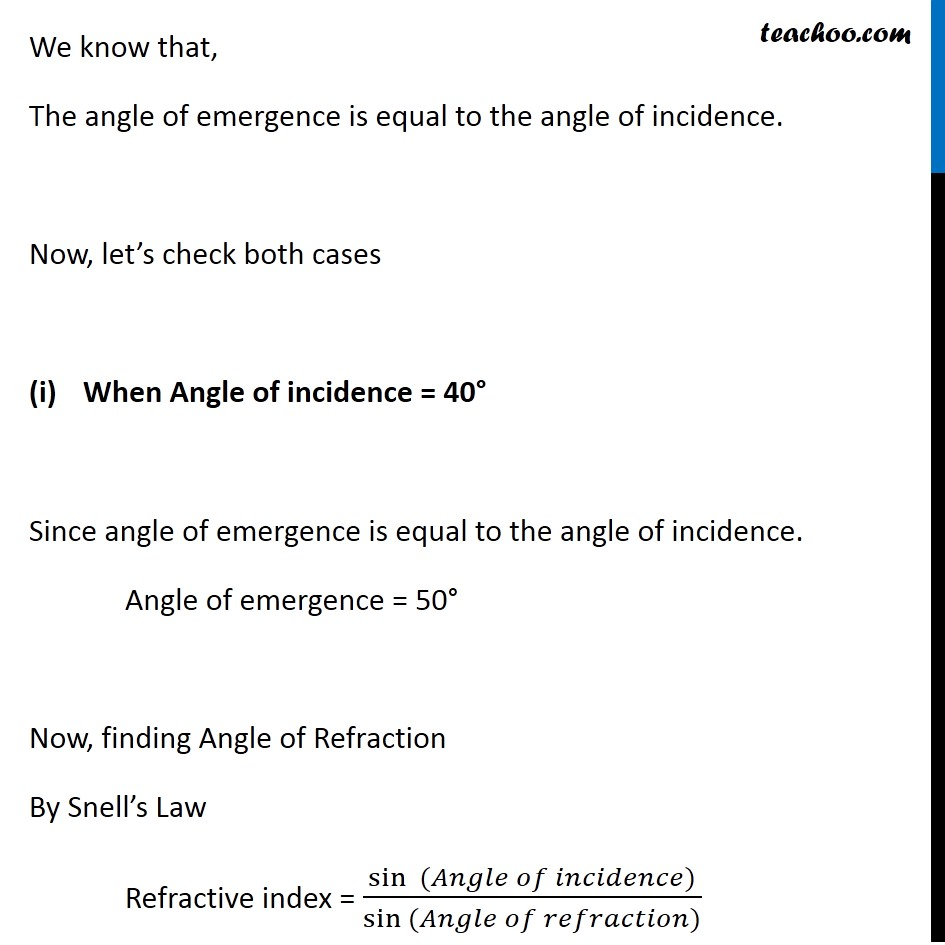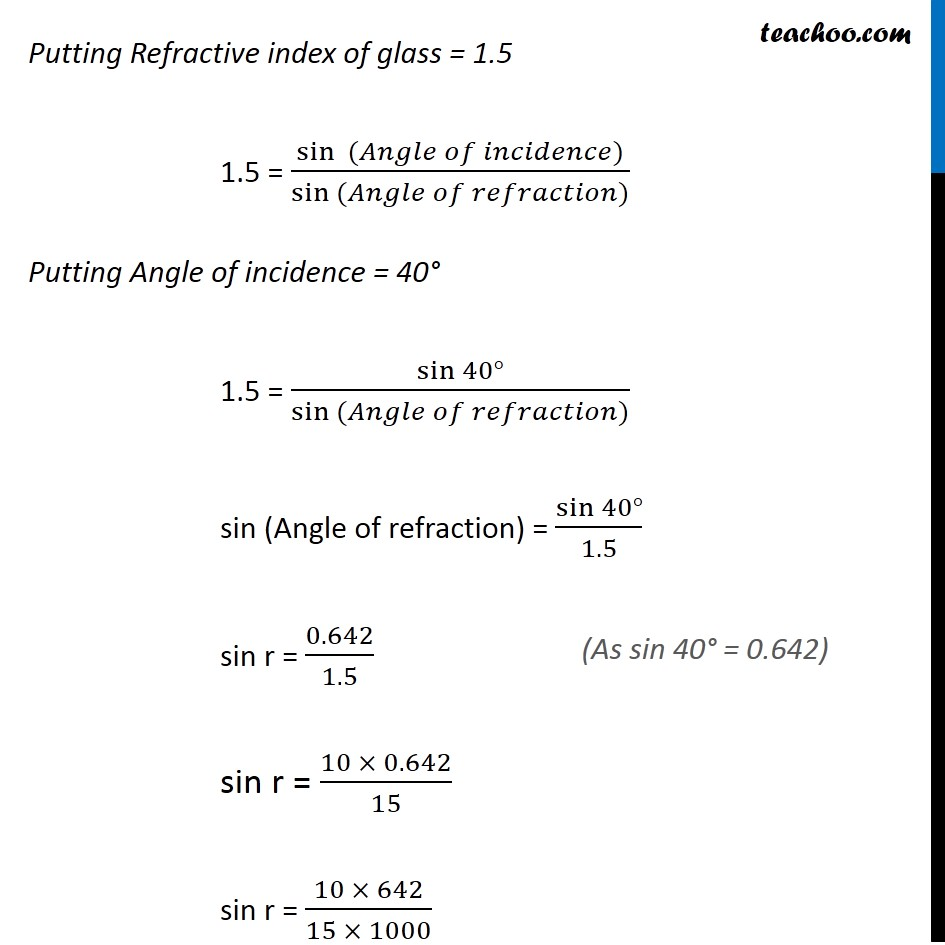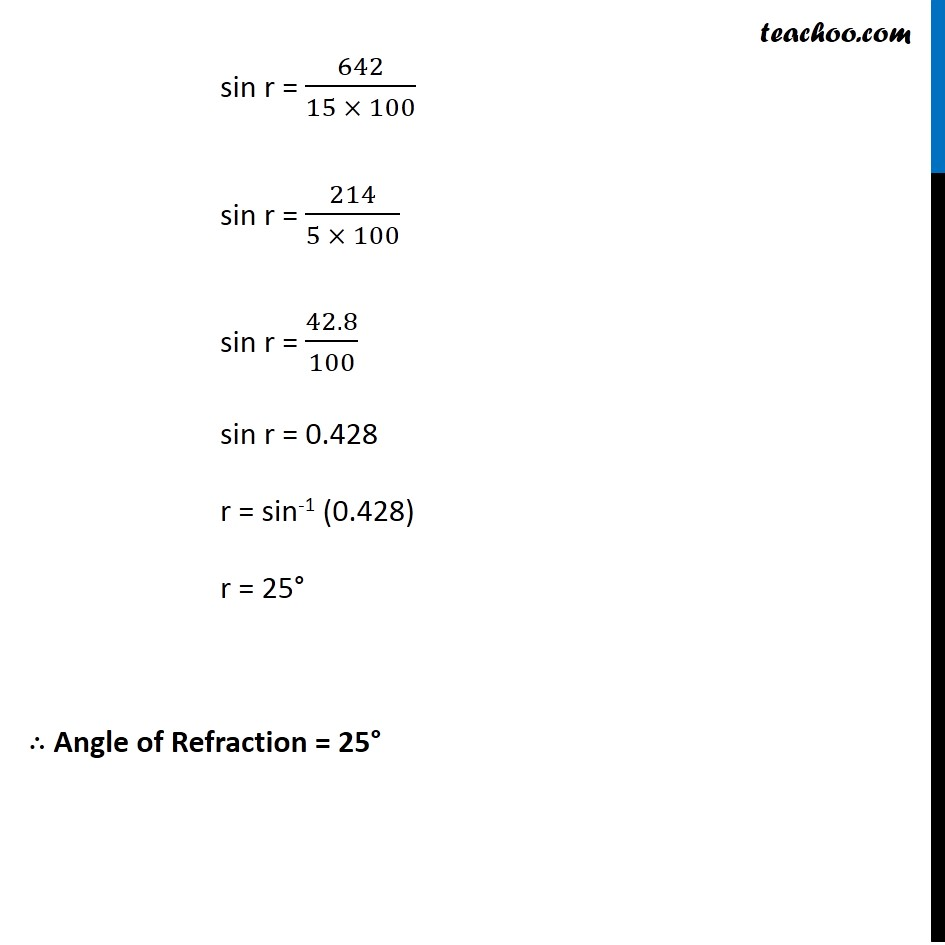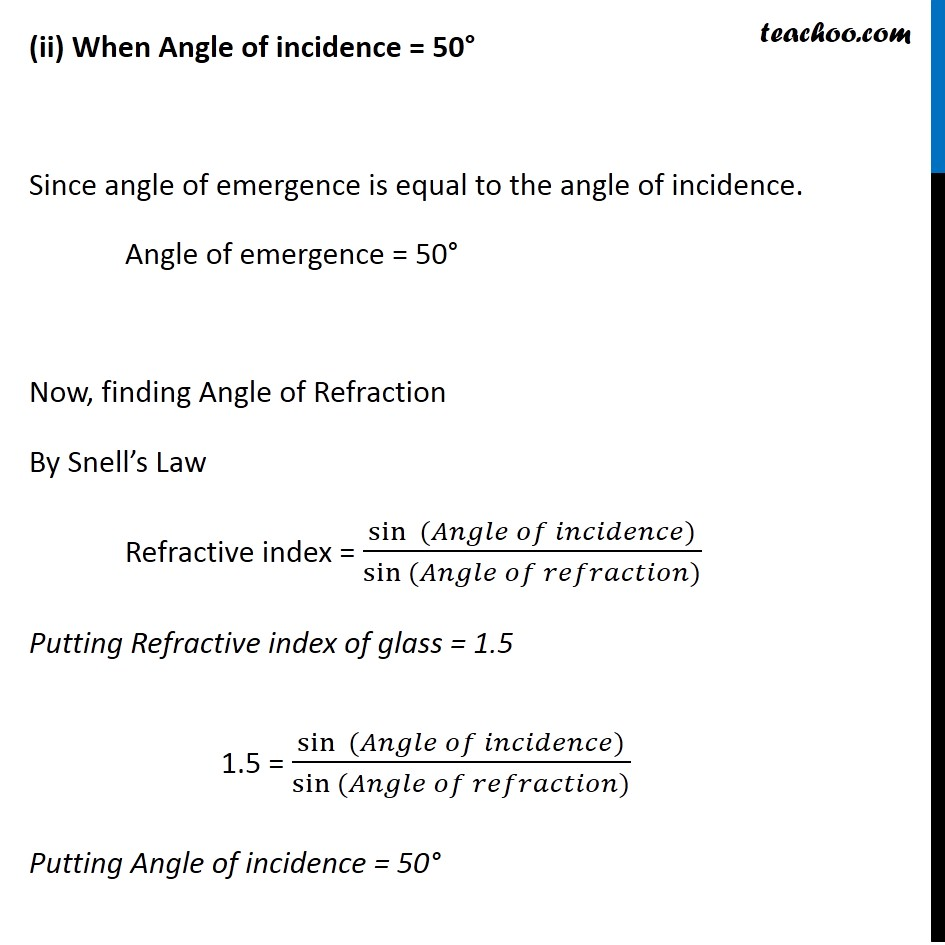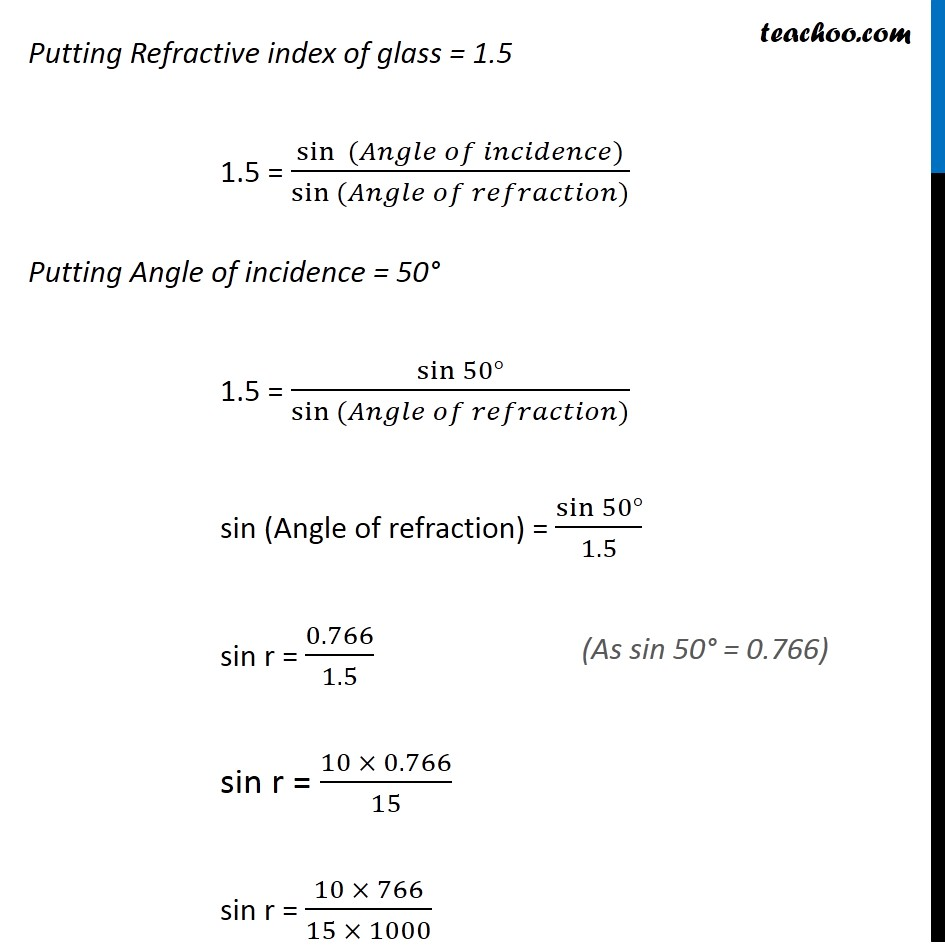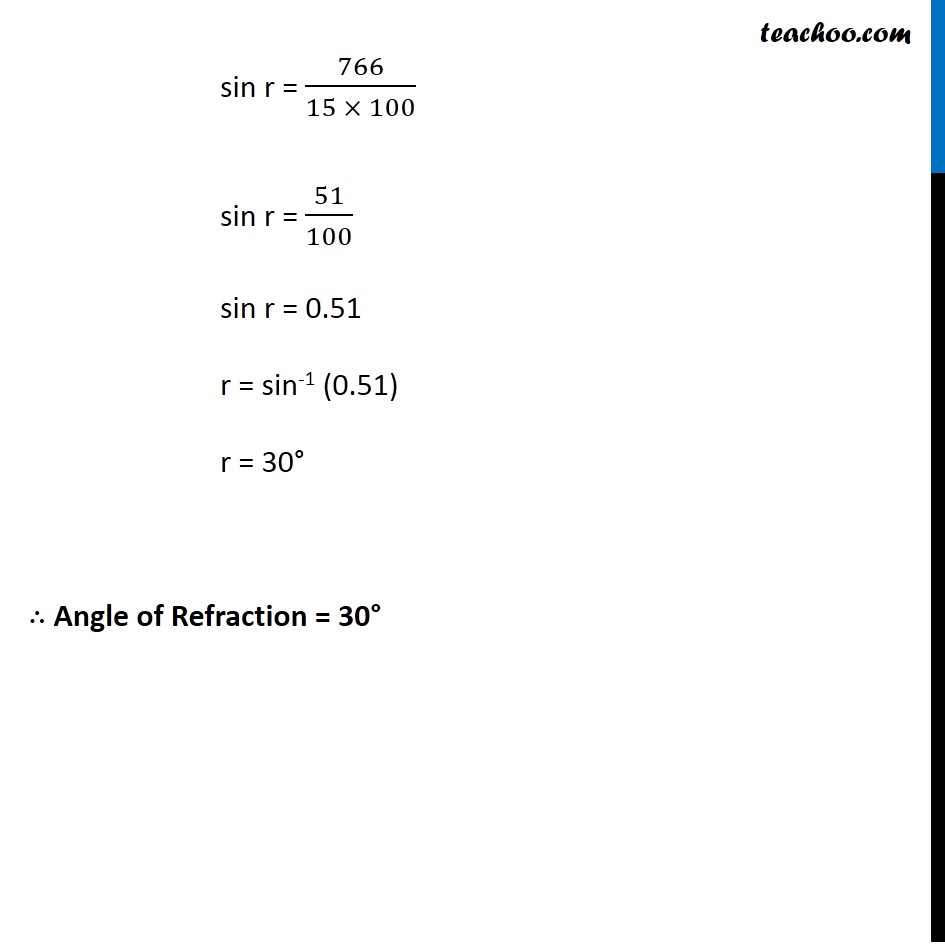## If the image formed by a spherical mirror for all positions of the object placed in front of it is always erect and diminished, what type of mirror is it?

Only a convex mirror always forms an erect and diminished image.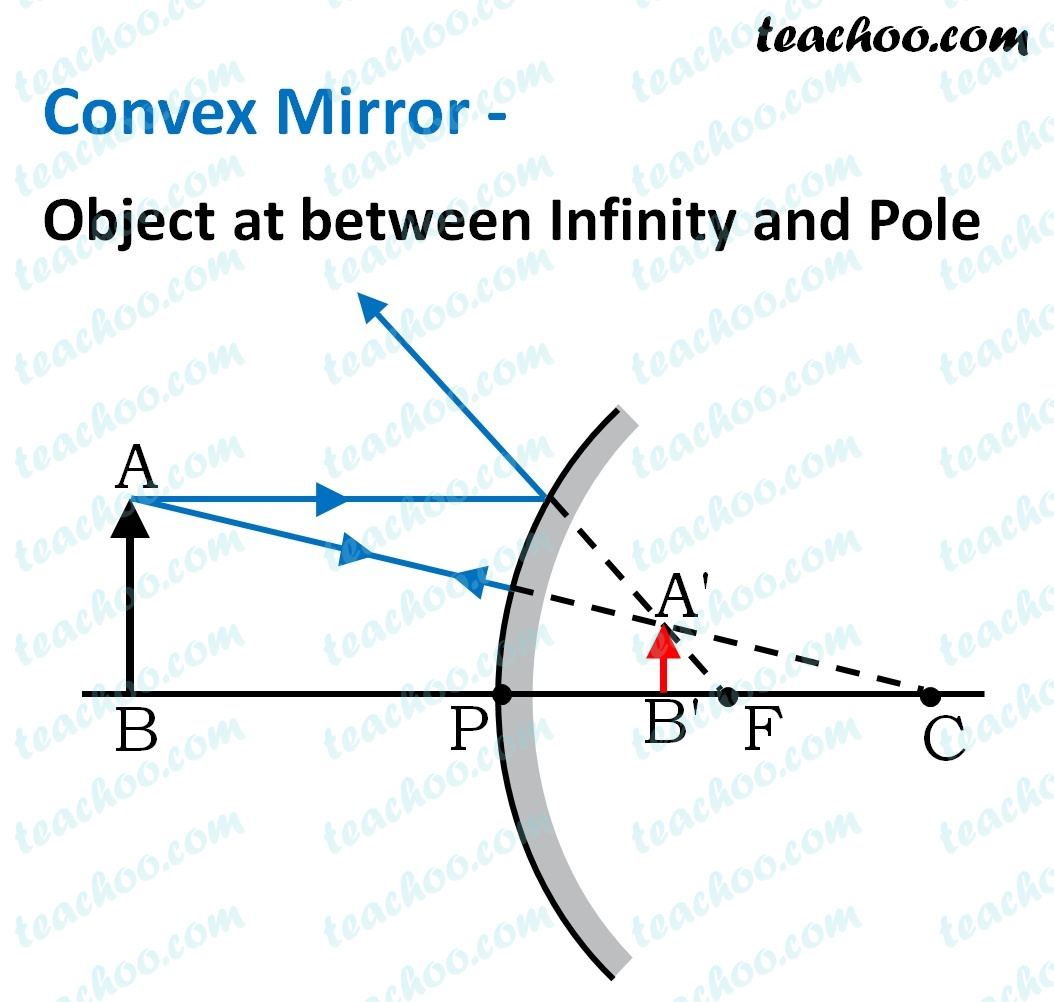## State the laws of refraction of light. Explain the term ‘absolute refractive index of a medium’ and write an expression to relate it with the speed of light in vacuum.

Laws of Refraction

The two laws of refraction of light are

1. Law 1
The Incident Ray,Refracted Ray and Normal all lie in Same plane
1. Law 2
The Ratio of sine of Angle of incidence and Angle of Refraction is constant for a Particular Medium.
This is also known as Snell’s Law.

Absolute Refractive Index of a Medium
The Refractive Index of a Medium with respect to vacuum is called Absolute Refractive Index.
The formula is
Refractive Index of a Medium in Vaccum = Speed of Light in Vaccum/Speed of Light in Medium

## Consider the path of a ray of light passing through a rectangular glass slab for different angles of incidence.

(i) Which one is greater: angle of incidence or angle of refraction?

(ii) What happens to the emergent angle on increasing the incident angle at air-glass interface?

(iii) State the conditions when no bending occurs.

(i) The ray of light enters from air to glass

Hence, it goes from rarer medium to denser medium.

As the light enters from rarer to denser medium, it moves towards the normal.

Hence, the angle of refraction will be less than the angle of incidence.

Angle of incidence will be greater.

(ii) We know that,

Angle of Emergence = Angle of Incidence

As we increase the angle of incidence, the angle of emergence will also keep on increasing.

(ii) There can be two conditions where no bending occurs

1. When ray of light falls perpendicular to the surface
i.e. when ray of light is parallel to the normal
2. When refractive index of both media are equal

## Position of screen = 74.0 cm

1. What is the focal length of the convex lens?
2. Where will the image be formed if he shifts the candle towards the lens at a position of 38 cm?
3. Draw a ray diagram to show the formation of the image in case (ii) as said above?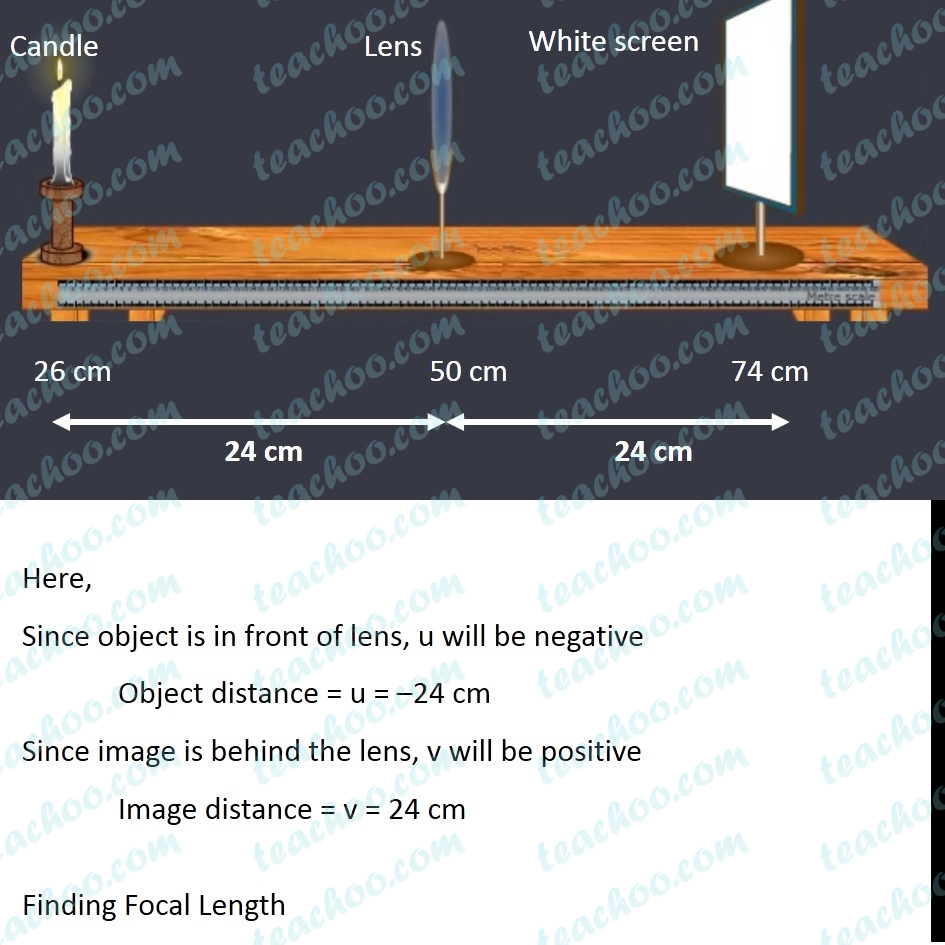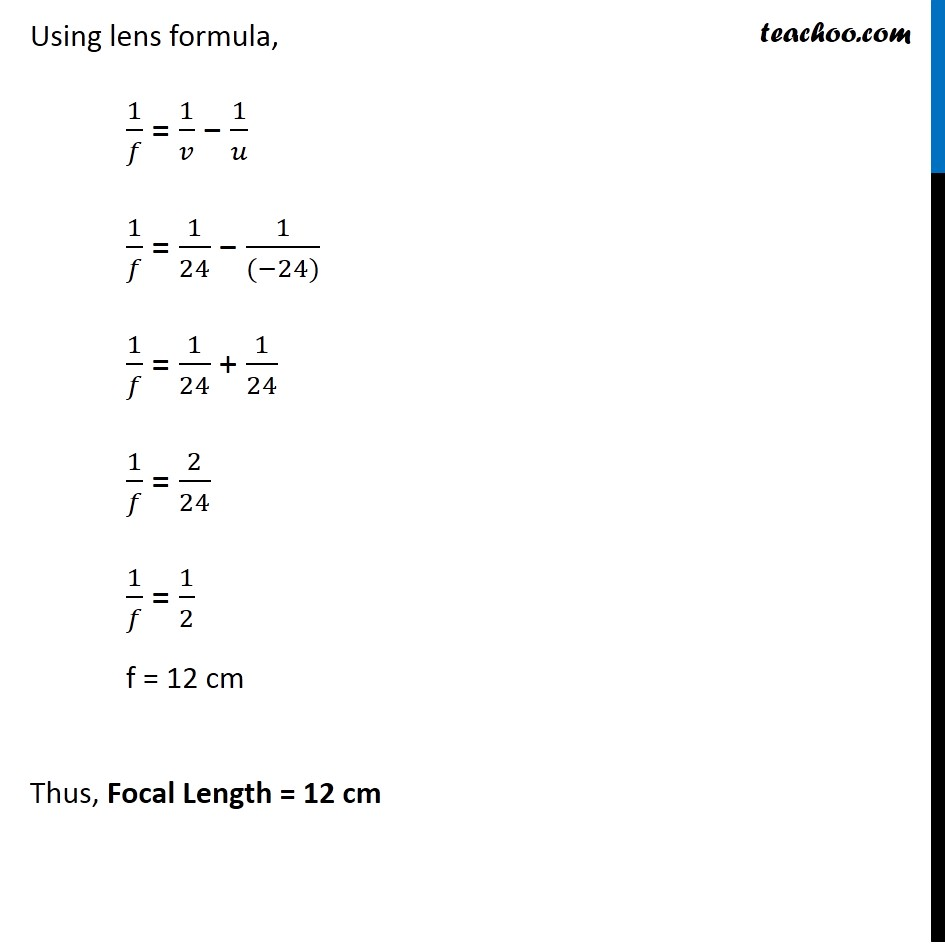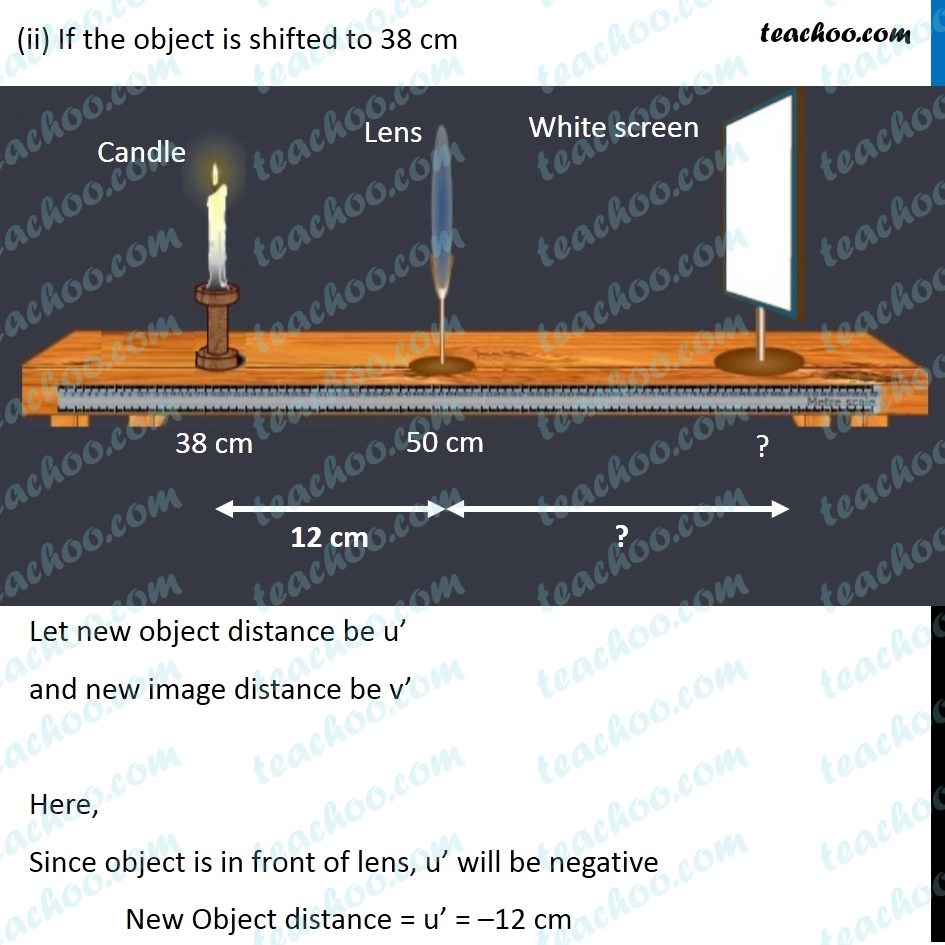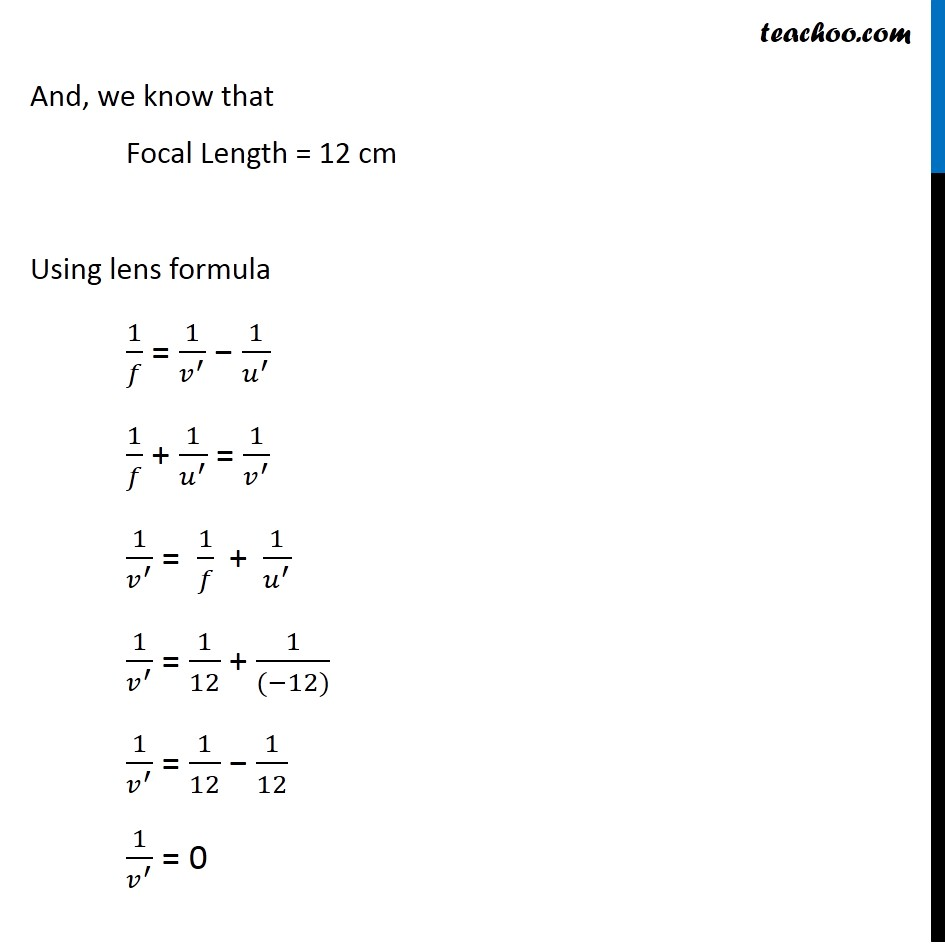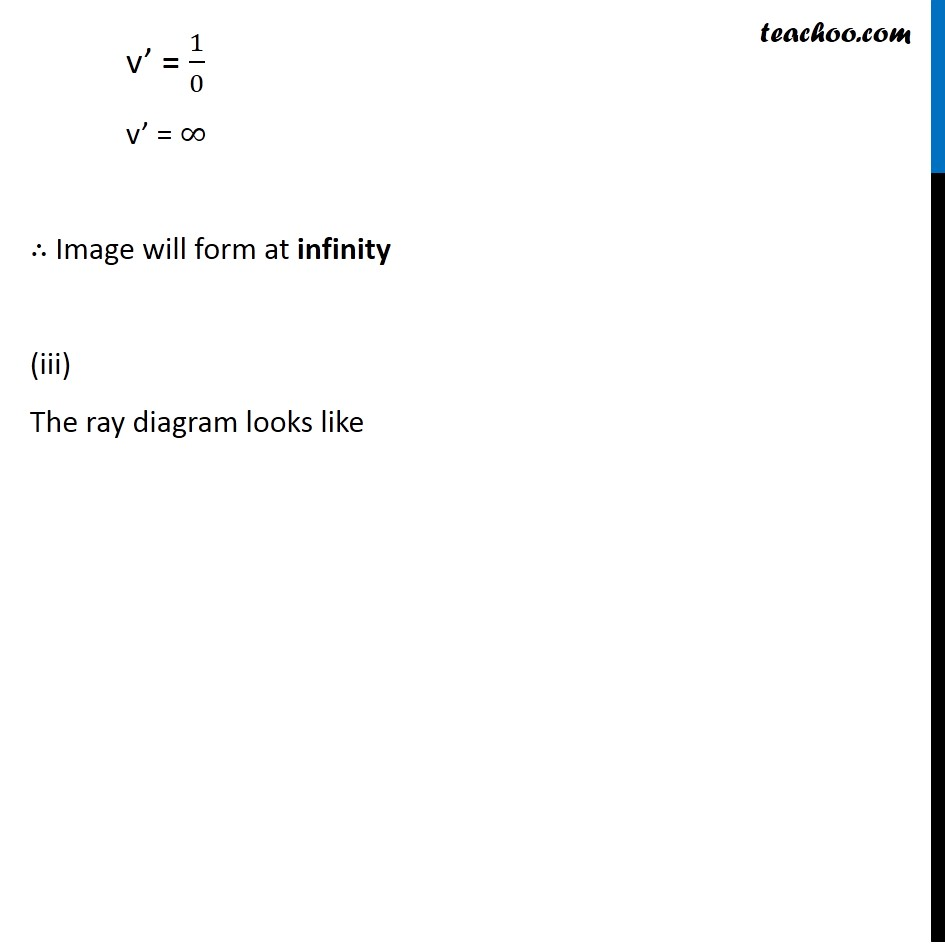Get live Maths 1-on-1 Classs - Class 6 to 12

### Transcript

Extra Question (3 Marks) - Question 1 Define power of a lens. What is its unit? One student uses a lens of focal length 50 cm and another of –50 cm. What is the nature of the lens and its power used by each of them? The power of a lens is defined as the reciprocal of its focal length. Power of a lens = 1/(𝐹𝑜𝑐𝑎𝑙 𝑙𝑒𝑛𝑔𝑡ℎ 𝑜𝑓 𝑡ℎ𝑒 𝑙𝑒𝑛𝑠) The SI Unit of Power is Dioptre. Note: SI unit of Power is Diopter when Focal length is measured in metres Focal length = 50 cm = 50/100 m = 1/2 m Power = 1/(𝐹𝑜𝑐𝑎𝑙 𝑙𝑒𝑛𝑔𝑡ℎ) = 1/(1/2) = 2 D Since focal length of the lens is positive, ∴ The lens is a convex lens. It is of converging nature. Focal length = −50 cm = (−50)/100 m = (−1)/2 m Power = 1/(𝐹𝑜𝑐𝑎𝑙 𝑙𝑒𝑛𝑔𝑡ℎ) = 1/(−1/2) = −2 D Since focal length of the lens is negative, ∴ The lens is a concave lens. It is of diverging nature. Extra Question (3 Marks) - Question 2 An object is placed at a distance of 60 cm from a concave lens of focal length 30 cm (i) Use lens formula to find the distance of the image from the lens Object is always placed in front of the lens Hence, object distance is negative Object distance = u = −60 cm Also, Focal length of a concave lens is negative Focal length = f = −30 cm Finding image distance Let the image distance = v Using lens formula, 1/𝑓 = 1/𝑣 − 1/𝑢 1/𝑓 + 1/𝑢 = 1/𝑣 1/𝑣 = 1/𝑓 + 1/𝑢 1/𝑣 = 1/((−30)) + 1/((−60)) 1/𝑣 = (−1)/30 − 1/60 1/𝑣 = (−2 − 1)/60 1/𝑣 = (−3)/60 𝑣 = (−60)/3 𝑣 = −20 cm Image is formed at a distance of 20 cm infront of the mirror We know that, The angle of emergence is equal to the angle of incidence. Now, let’s check both cases When Angle of incidence = 40° Since angle of emergence is equal to the angle of incidence. Angle of emergence = 50° Now, finding Angle of Refraction By Snell’s Law Refractive index = sin⁡〖 (𝐴𝑛𝑔𝑙𝑒 𝑜𝑓 𝑖𝑛𝑐𝑖𝑑𝑒𝑛𝑐𝑒)〗/〖sin 〗⁡〖(𝐴𝑛𝑔𝑙𝑒 𝑜𝑓 𝑟𝑒𝑓𝑟𝑎𝑐𝑡𝑖𝑜𝑛)〗 Putting Refractive index of glass = 1.5 1.5 = sin⁡〖 (𝐴𝑛𝑔𝑙𝑒 𝑜𝑓 𝑖𝑛𝑐𝑖𝑑𝑒𝑛𝑐𝑒)〗/〖sin 〗⁡〖(𝐴𝑛𝑔𝑙𝑒 𝑜𝑓 𝑟𝑒𝑓𝑟𝑎𝑐𝑡𝑖𝑜𝑛)〗 Putting Angle of incidence = 40° 1.5 = sin⁡〖40°〗/〖sin 〗⁡〖(𝐴𝑛𝑔𝑙𝑒 𝑜𝑓 𝑟𝑒𝑓𝑟𝑎𝑐𝑡𝑖𝑜𝑛)〗 sin (Angle of refraction) = sin⁡〖40°〗/1.5 sin r = 0.642/1.5 sin r = (10 × 0.642)/15 sin r = (10 × 642)/(15 × 1000) sin r = 642/(15 × 100) sin r = 214/(5 × 100) sin r = 42.8/100 sin r = 0.428 r = sin-1 (0.428) r = 25° ∴ Angle of Refraction = 25° (ii) When Angle of incidence = 50° Since angle of emergence is equal to the angle of incidence. Angle of emergence = 50° Now, finding Angle of Refraction By Snell’s Law Refractive index = sin⁡〖 (𝐴𝑛𝑔𝑙𝑒 𝑜𝑓 𝑖𝑛𝑐𝑖𝑑𝑒𝑛𝑐𝑒)〗/〖sin 〗⁡〖(𝐴𝑛𝑔𝑙𝑒 𝑜𝑓 𝑟𝑒𝑓𝑟𝑎𝑐𝑡𝑖𝑜𝑛)〗 Putting Refractive index of glass = 1.5 1.5 = sin⁡〖 (𝐴𝑛𝑔𝑙𝑒 𝑜𝑓 𝑖𝑛𝑐𝑖𝑑𝑒𝑛𝑐𝑒)〗/〖sin 〗⁡〖(𝐴𝑛𝑔𝑙𝑒 𝑜𝑓 𝑟𝑒𝑓𝑟𝑎𝑐𝑡𝑖𝑜𝑛)〗 Putting Angle of incidence = 50° Putting Refractive index of glass = 1.5 1.5 = sin⁡〖 (𝐴𝑛𝑔𝑙𝑒 𝑜𝑓 𝑖𝑛𝑐𝑖𝑑𝑒𝑛𝑐𝑒)〗/〖sin 〗⁡〖(𝐴𝑛𝑔𝑙𝑒 𝑜𝑓 𝑟𝑒𝑓𝑟𝑎𝑐𝑡𝑖𝑜𝑛)〗 Putting Angle of incidence = 50° 1.5 = sin⁡〖50°〗/〖sin 〗⁡〖(𝐴𝑛𝑔𝑙𝑒 𝑜𝑓 𝑟𝑒𝑓𝑟𝑎𝑐𝑡𝑖𝑜𝑛)〗 sin (Angle of refraction) = sin⁡〖50°〗/1.5 sin r = 0.766/1.5 sin r = (10 × 0.766)/15 sin r = (10 × 766)/(15 × 1000) (As sin 50° = 0.766) sin r = 766/(15 × 100) sin r = 51/100 sin r = 0.51 r = sin-1 (0.51) r = 30° ∴ Angle of Refraction = 30° Here, Since object is in front of lens, u will be negative Object distance = u = –24 cm Since image is behind the lens, v will be positive Image distance = v = 24 cm Finding Focal Length Using lens formula, 1/𝑓 = 1/𝑣 − 1/𝑢 1/𝑓 = 1/24 − 1/((−24)) 1/𝑓 = 1/24 + 1/24 1/𝑓 = 2/24 1/𝑓 = 1/2 f = 12 cm Thus, Focal Length = 12 cm (ii) If the object is shifted to 38 cm Let new object distance be u’ and new image distance be v’ Here, Since object is in front of lens, u’ will be negative New Object distance = u’ = –12 cm And, we know that Focal Length = 12 cm Using lens formula 1/𝑓 = 1/𝑣^′ − 1/𝑢^′ 1/𝑓 + 1/𝑢^′ = 1/𝑣^′ 1/𝑣^′ = 1/𝑓 " + " 1/𝑢^′ 1/𝑣^′ = 1/12 + 1/((−12)) 1/𝑣^′ = 1/12 − 1/12 1/𝑣^′ = 0 v’ = 1/0 v’ = ∞ ∴ Image will form at infinity (iii) The ray diagram looks like